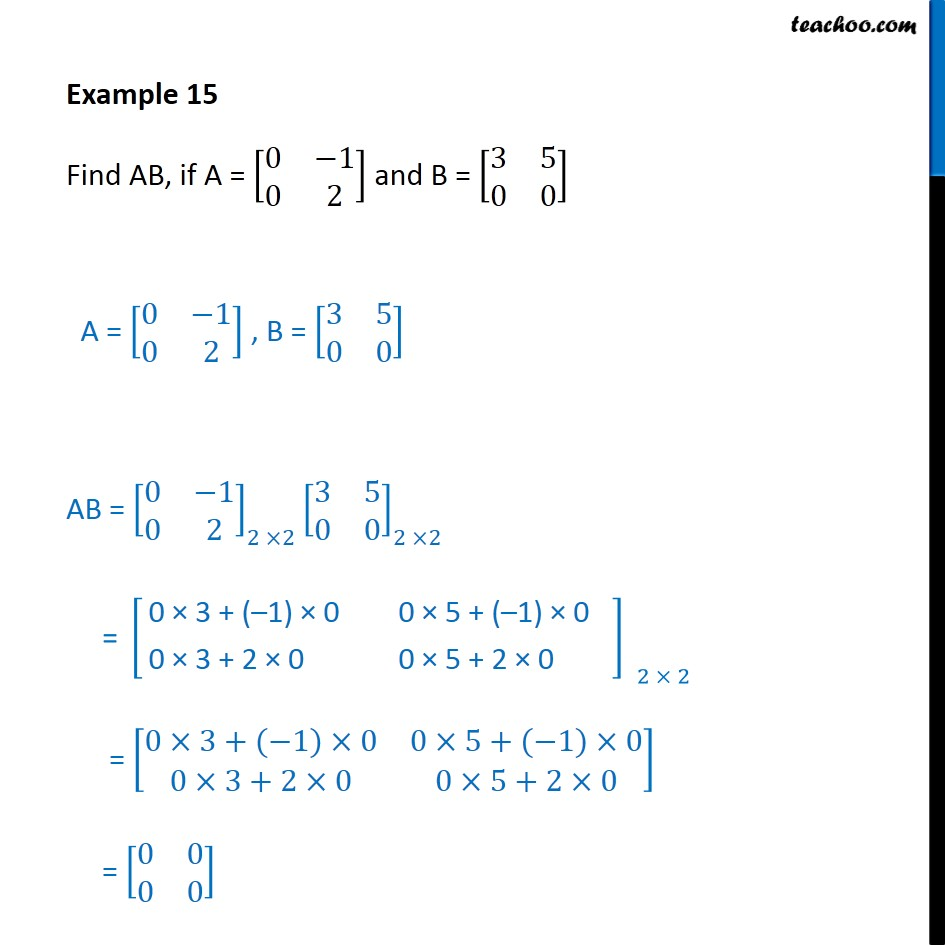Learn All Concepts of Chapter 3 Class 12 Matrices - FREE. Check - Matrices Class 12 - Full video1. Chapter 3 Class 12 Matrices
2. Serial order wise
3. Examples

Transcript

Example 15 Find AB, if A = [■8(0&−1@0&2)] and B = [■8(3&5@0&0)] A = [■8(0&−1@0&2)] , B = [■8(3&5@0&0)] AB = [■8(0&−1@0&2)]_(2 ×2) [■8(3&5@0&0)]_(2 ×2) = [■8(0×3+(−1)×0&0×5+(−1)×0@0×3+2×0&0×5+2×0)] = [■8(0&0@0&0)]

Examples

Chapter 3 Class 12 Matrices
Serial order wise